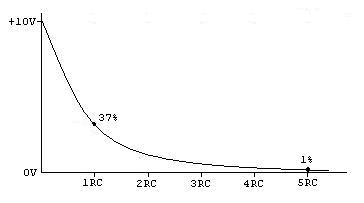# The symbol t in the capacitor discharge formula q= (q0e)^(–t/RC)

hidemi
Homework Statement:
In the capacitor discharge formula q= (q0e)^(–t/RC) the symbol t represents:
A)the time constant
B)the time it takes for C to lose the fraction 1/e of its initial charge
C)the time it takes for C to lose the fraction (1 – 1/e) of its initial charge
D)the time it takes for C to lose essentially all of its initial charge
E)none of the above
Relevant Equations:
q= (q0e)^(–t/RC)
If none of the above is correct, what is a good definition of the symbol t (time)?

Homework Helper
Gold Member
Clock time. It's the same ##t## as in ##x=v_0t+\frac{1}{2}at^2##. Here the imaginary clock starts ticking at ##t=0## when the capacitor starts discharging.

•rsk and hidemi
hidemi
Clock time. It's the same ##t## as in ##x=v_0t+\frac{1}{2}at^2##. Here the imaginary clock starts ticking at ##t=0## when the capacitor starts discharging.
Ok I see.
Another question is that why we need to divide the time by the time constant (tao)?

Homework Helper
Gold Member
Ok I see.
Another question is that why we need to divide the time by the time constant (tao)?
As you know, the charge on the capacitor at any time ##t## is given by ##q(t)=q_0e^{-\frac{t}{RC}}.## The product ##RC## has dimensions of time. So we define time constant ##\tau=RC## and substitute in the equation to get ##q(t)=q_0e^{-\frac{t}{\tau}}.##

•hidemi
hidemi
As you know, the charge on the capacitor at any time ##t## is given by ##q(t)=q_0e^{-\frac{t}{RC}}.## The product ##RC## has dimensions of time. So we define time constant ##\tau=RC## and substitute in the equation to get ##q(t)=q_0e^{-\frac{t}{\tau}}.##
Ok I see. Thank you.

•berkeman and kuruman
Mentor
Another helpful mental picture is to understand that for each time constant ##\tau## the level of charge goes down to ##\frac{1}{e}## of its initial level Q. That is about 37% left after each time constant. So after one time constant Q goes to Q*0.37Q, after 2 time constants, Q has gone down to Q*(0.37)^2, and so on.https://www.eecs.tufts.edu/~dsculley/tutorial/rc/dischargeCurve.jpg

•hidemi and Delta2